# 8. a. Prove that f(x) = cos x is continuous on R. b. If ECR and...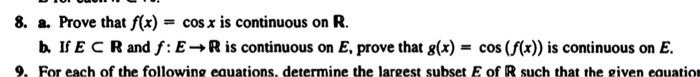8. a. Prove that f(x) = cos x is continuous on R. b. If ECR and f: E R is continuous on E, prove that 8(x) = cos (f(x)) is continuous on E. 9. For each of the following equations, determine the largest subset E of R such that the given equation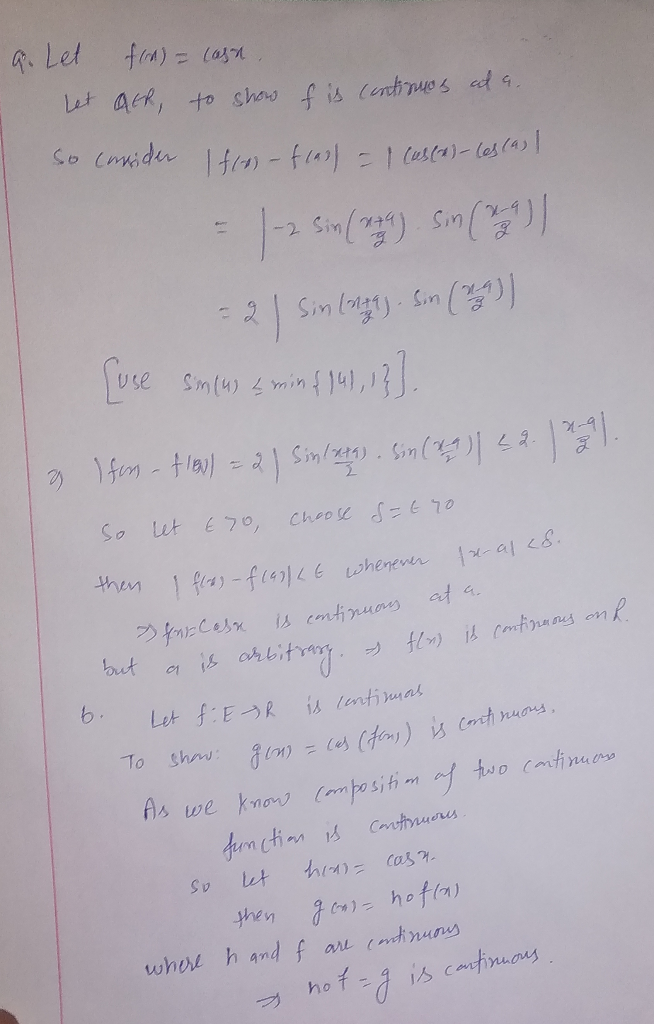##### Add Answer of: 8. a. Prove that f(x) = cos x is continuous on R. b. If ECR and...
Similar Homework Help Questions
• ### S f(r)da= g(x)dz. Prove a,bsuch that (8) Suppose f and g are continuous functions on that...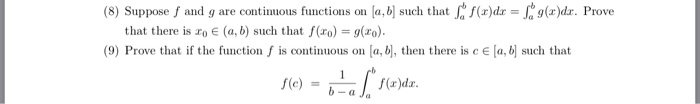S f(r)da= g(x)dz. Prove a,bsuch that (8) Suppose f and g are continuous functions on that there is ro e (a, b) such that f(ro) = g(xo). (9) Prove that if the function f is continuous on a, b, then there is c E [a, b such that f(x)dax a Ja f(e) S f(r)da= g(x)dz. Prove a,bsuch that (8) Suppose f and g are continuous functions on that there is ro e (a, b) such that f(ro) = g(xo). (9)...

• ### continuous function |f(x)|

Let A be a subset of R, let f: A --> R and let |f| be defined by|f|(x) := |f(x)| for x in A.1. Prove if f is continuous at a point c in A, then |f| iscontinuous at c2. Prove if f is continuous on A, then |f| is continuous on A.

• ### 3. Let f, g : [a,b] → R be functions such that f is integrable, g is continuous, and g(x) >0 for ...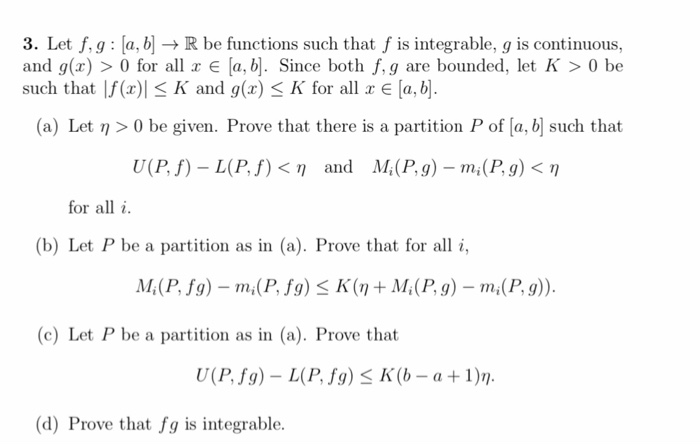3. Let f, g : [a,b] → R be functions such that f is integrable, g is continuous, and g(x) >0 for all r E [a, b] Since both f,g are bounded, let K >0 be such that lf(z)| K and g(x) K for all x E [a3] (a) Let n > 0 be given. Prove that there is a partition P of [a, b such that U (P. f) _ L(P./) < η and Mi(P4)-mi(P4) < η for all...

• ### A polar curve r = f() has parametric equations x = f(0) cos(8), y = f(0)...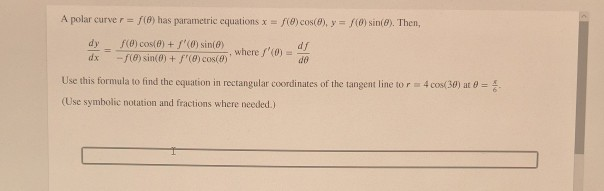A polar curve r = f() has parametric equations x = f(0) cos(8), y = f(0) sin(8). Then, dy f() cos(0) + f (0) sin(e) d/ where / --f(8) sin(0) + / (8) cos(8) do Use this formula to find the equation in rectangular coordinates of the tangent line to r = 4 cos(30) at 0 = (Use symbolic notation and fractions where needed.)

• ### continuous function sqrt(f(x))

Let A be a subset of R, let f : A --> R and let f(x) >= 0 forall x in A. Let √fbe defined for x in A by(√f)(x) := √f(x)1. Prove if f is continuous at a point c in A, then √f iscontinuous at c2. Prov if f is continuous on A, then √f is continuous onA.

• ### Prove f is continuousLet A be non-empty subset of a metric space (X,d). Define thefunction by . Prove that f is continuous.

• ### Prove that there is no continuous bijection from the unit circle S1 = y21 onto any subset of R. (x,y) E R2 Prove that there is no continuous bijection from the unit circle S1 = y21 onto any subs...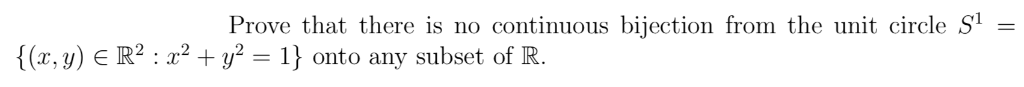Prove that there is no continuous bijection from the unit circle S1 = y21 onto any subset of R. (x,y) E R2 Prove that there is no continuous bijection from the unit circle S1 = y21 onto any subset of R. (x,y) E R2

• ### Suppose f is a continuous on R and f(x + y) = f(x) + f(y) for...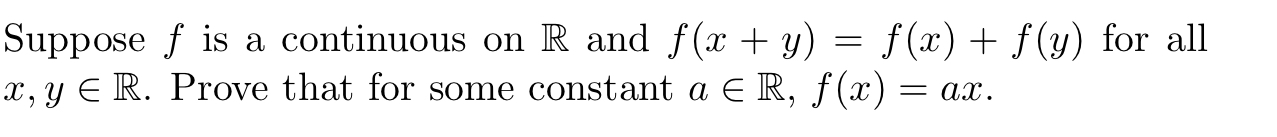Suppose f is a continuous on R and f(x + y) = f(x) + f(y) for all x, y ∈ R. Prove that for some constant a ∈ R, f(x) = ax. Suppose f is a continuous on R and f(x + y) = f(x) + f(y) for all X, Y E R. Prove that for some constant a ER, f(x) = ax.

• ### 3. Let f, g : a, b] → R be functions such that f is integrable, g is continuous. and g(x) 〉 0 fo...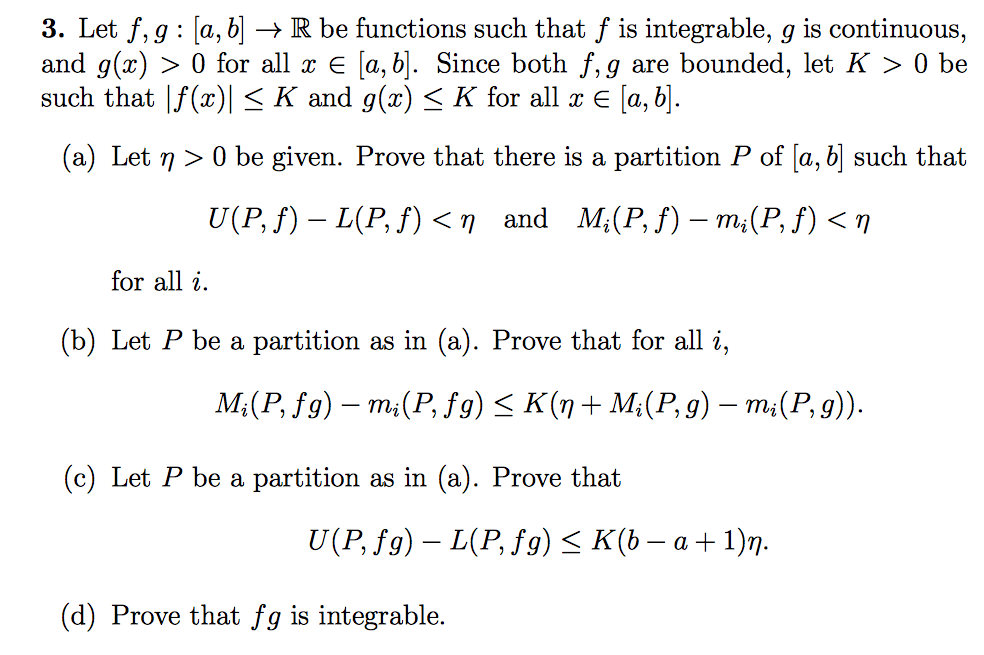3. Let f, g : a, b] → R be functions such that f is integrable, g is continuous. and g(x) 〉 0 for all x є a,b]. Since both f, g are bounded, let K 〉 0 be such that |f(x) K and g(x) < K for all x E [a,b (a) Let n > 0 be given. Prove that there is a partition P of [a, b such that for all i 2. (b) Let P be a...

• ### Let the function f: (a, b) → R is continuous in (a, b). If sup {f(x):...

Let the function f: (a, b) → R is continuous in (a, b). If sup {f(x): x ∈ (a, b)} = L> 0 and inf {f(x): x ∈ (a, b)} = M <0, then prove that there is a c ∈ (a , b) such that f (c) = 0.

Free Homework App